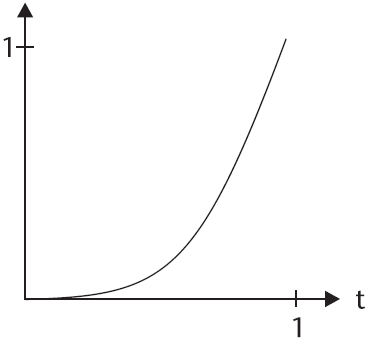# 创建自定义的jQuery补间动画运动函数及其实现的数学原理发布者 newghost  发布时间 1429888963890

jQuery动画可以用animte函数实现，该方法通过CSS样式将元素从一个状态改变为另一个状态。

### animte函数

CSS属性值是逐渐改变的，这样就可以创建动画效果。只有数字值可创建动画，比如：

`\$("#content").animate({height:"100px"});`

```\$(selector).animate(styles,options)
\$(selector).animate(styles,speed,easing,callback)```

• speed - 设置动画的速度
• easing - 规定要使用的 easing 函数
• callback - 规定动画完成之后要执行的函数
• step - 规定动画的每一步完成之后要执行的函数
• queue - 布尔值。指示是否在效果队列中放置动画。如果为 false，则动画将立即开始
• specialEasing - 来自 styles 参数的一个或多个 CSS 属性的映射，以及它们的对应 easing 函数

`\$("#content").animate({height:"100px"}, "linear");`

### easing函数

jQuery1.4的linear和swing的动画实现代码是这样的，最新版的jQuery做了改进，只使用了一个参数p，但不容易看出各个参数的作用，因此这里使用了一个比较原始版本。

`jQuery.extend({    ……    easing: {        linear: function( p, n, firstNum, diff ) {            return firstNum + diff * p;        },        swing: function( p, n, firstNum, diff ) {            return ((-Math.cos(p*Math.PI)/2) + 0.5) * diff + firstNum;        }    },    ……});`

t：time, b: begin, c: change, d: duration

t: current_time  当前时间
b: start_value   初始位置值（比如x轴运行时）
c: end_value     change in position (单位时间内的改变值）
d: total_time    持续时间

`jQuery.easing.method(    null†,    current_time,    start_value,    end_value,    total_time)`x 轴代表时间t (单位缩放为1)
y 轴代表距离

`jQuery.easing['easeInQuint'] = function(p, t, b, c, d) {    return c * Math.pow (t/d, 4) + b;};//应用： 将当前页面按照easeInQuint运动趋势滚动到项部\$('html, body').animate({    scrollTop: 0}, {    duration : 500  , easing   : 'easeInQuint'})`

`jQuery.easing['easeOutBounce'] = function (x, t, b, c, d) {    if ((t/=d) < (1/2.75)) {        return c*(7.5625*t*t) + b;    } else if (t < (2/2.75)) {        return c*(7.5625*(t-=(1.5/2.75))*t + .75) + b;    } else if (t < (2.5/2.75)) {        return c*(7.5625*(t-=(2.25/2.75))*t + .9375) + b;    } else {        return c*(7.5625*(t-=(2.625/2.75))*t + .984375) + b;    }}\$('.navbar.navbar-inverse').animate({    top: 200}, {    duration : 1000  , easing   : 'easeOutBounce'})`

##### 开源的 OurJS
OurJS开源博客已经迁移到 OnceOA 平台。

##### 关注我们

OnceJS